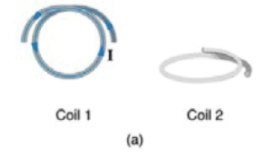# Problem: Consider the coils depicted in the figure. What is the value of the magnetic flux at coil 2 due to coil 1, which has a current I passing through it?

###### FREE Expert Solution

Magnetic flux through a coil:

$\overline{){\mathbf{\varphi }}{\mathbf{=}}{\mathbf{B}}{\mathbf{·}}{\mathbf{A}}}$

87% (318 ratings)###### Problem Details

Consider the coils depicted in the figure.What is the value of the magnetic flux at coil 2 due to coil 1, which has a current I passing through it?

Frequently Asked Questions

What scientific concept do you need to know in order to solve this problem?

Our tutors have indicated that to solve this problem you will need to apply the Magnetic Flux concept. You can view video lessons to learn Magnetic Flux. Or if you need more Magnetic Flux practice, you can also practice Magnetic Flux practice problems.

What professor is this problem relevant for?

Based on our data, we think this problem is relevant for Professor Puneet's class at CLEMSON.